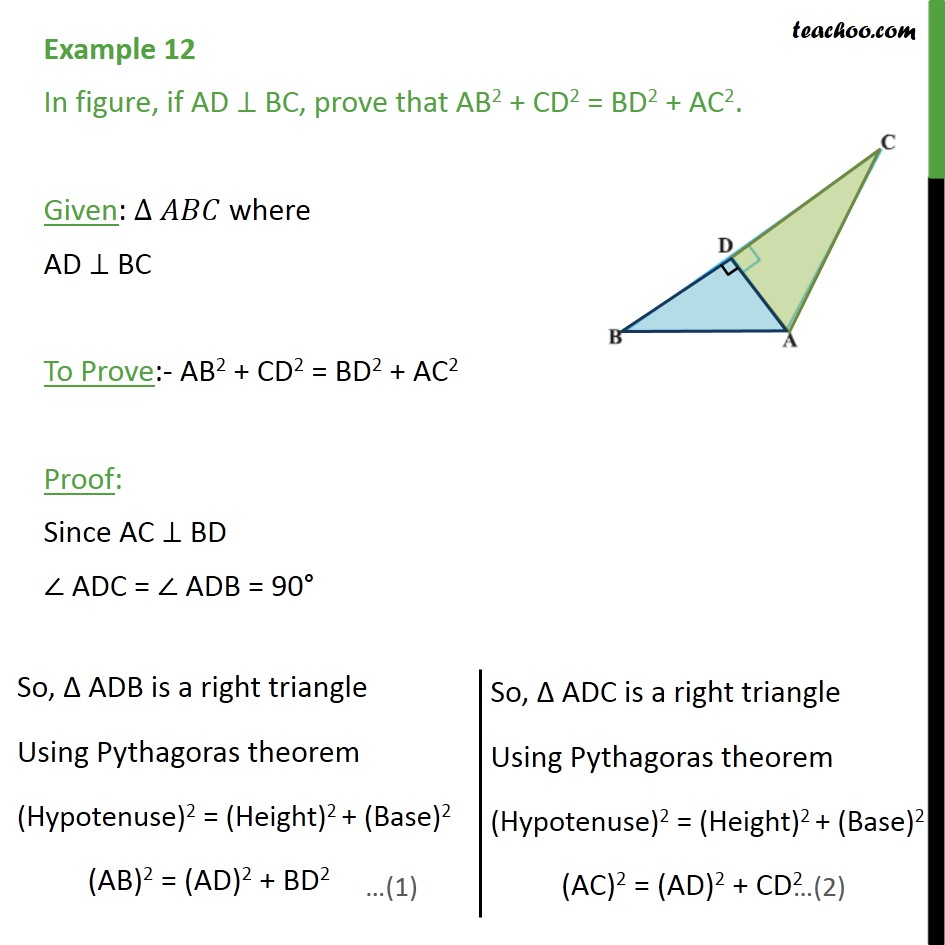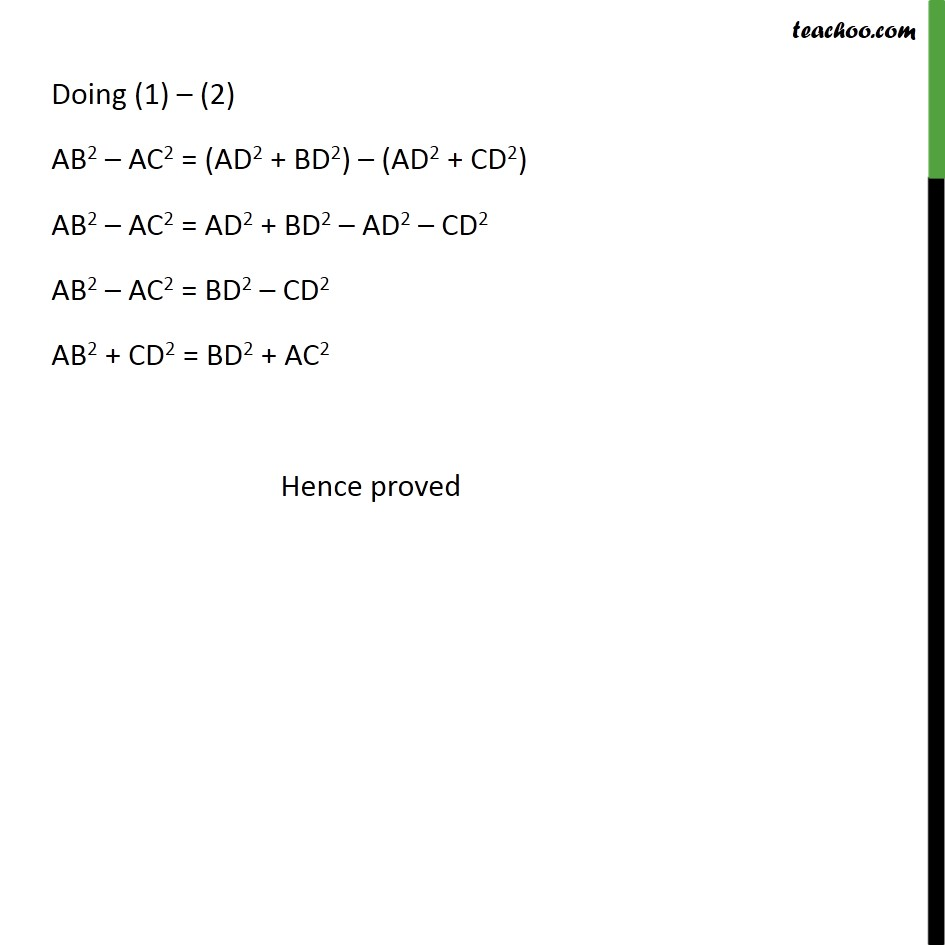1. Chapter 6 Class 10 Triangles
2. Concept wise
3. Pythagoras Theoram - Proving

Transcript

Example 12 In figure, if AD BC, prove that AB2 + CD2 = BD2 + AC2. Given: where AD BC To Prove:- AB2 + CD2 = BD2 + AC2 Proof: Since AC BD ADC = ADB = 90 Doing (1) (2) AB2 AC2 = (AD2 + BD2) (AD2 + CD2) AB2 AC2 = AD2 + BD2 AD2 CD2 AB2 AC2 = BD2 CD2 AB2 + CD2 = BD2 + AC2 Hence proved

Pythagoras Theoram - Proving